#DAY 29
1
Software Development

## 寫在前面

• queue
• stack
• tree
• binary tree
• hash table
等等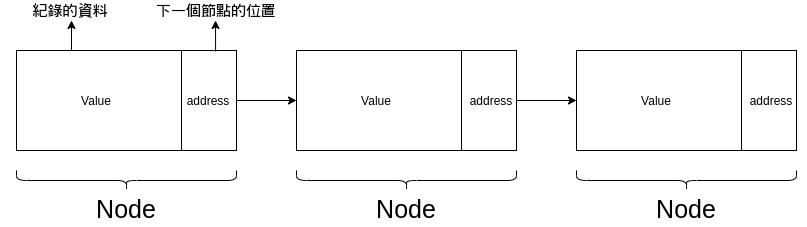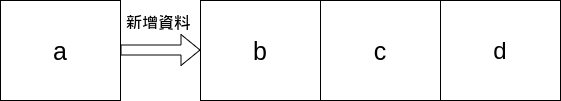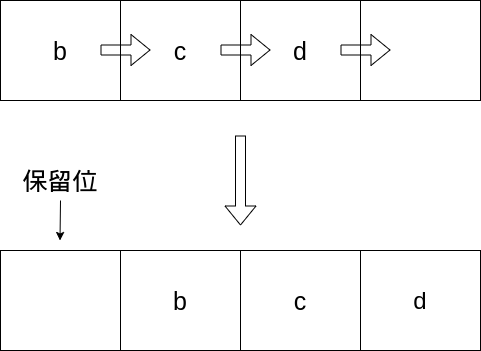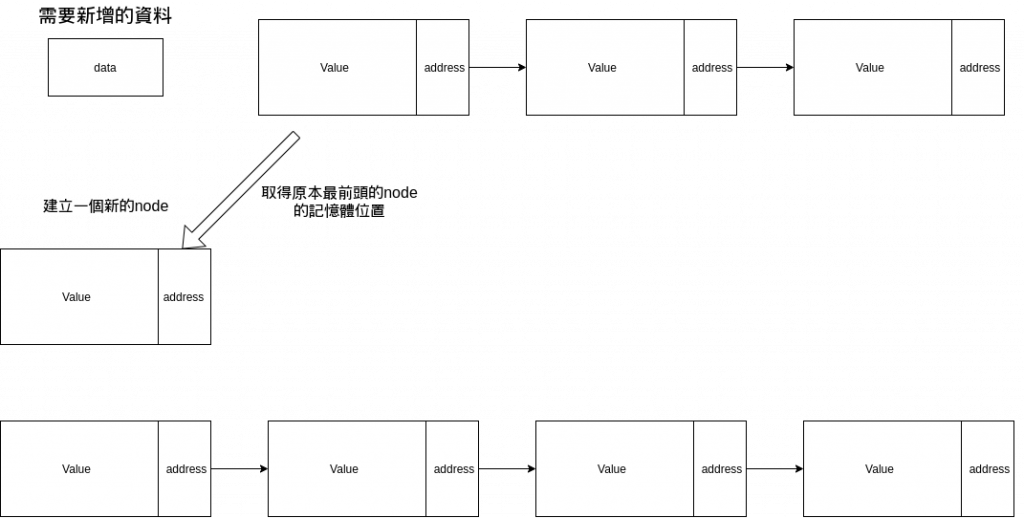## Queue

FIFO

Queue最大的特性就是first in first out### 結構

``````type node struct {
value interface{}
next  *node
}

type Queue struct {
rear   *node
length int
}
``````

node具有兩個資料,一個是真正的儲存資料value,這裡使用golang的interface作為動態型別
next則是一個指標,指向下一個node的位置

Queue表示的是整個queue的結構,這裡你需要頭跟尾的指標,你才能針對頭跟尾去做移除跟添加的動作

### 方法

queue至少需要幾個方法

• Empty() 用來判斷這個queue是否為空
• Push() 用來增加元素
• Peek() 用來確認頭元素
• Get() 取出頭元素(從queue中移除)
``````func (q *Queue) Empty() bool {
return q.length == 0 //使用內建的length屬性來判斷是否為空
}

func (q *Queue) Push(value interface{}) {
newnode := &node{value, nil} //先為使用者的輸入值建立node
if q.Empty() { //如果原本是空的則將頭尾指向同一個node
q.rear = newnode
} else {
q.rear.next = newnode //否則將原本node的尾巴指向新的node
q.rear = newnode //並且將queue的尾巴指向新的node
}
q.length++ //減少length的值
}

func (q *Queue) Peek() interface{} {
if q.Empty() { //若為空則回傳nil
return nil
}
}

func (q *Queue) Get() interface{} {
if q.Empty() { //若為空則回傳nil
return nil
}
defer func() { temp = nil }() //這邊設定Get結束時要將取出的資料初始化
if temp.next == nil { //如果下一個node為空(也就是現在會取出最後一個) 則將head及rear初始化
q.rear = nil
} else {
}
q.length-- //減少length的值

return temp.value
}

func (q *Queue) Prt() { //這邊是多餘的方法,用來印出所有的元素
if !q.Empty() {
fmt.Print(temp.value)
for temp.next != nil { //如果下一個元素不為空則印出下一個元素
fmt.Print(temp.next.value)
temp = temp.next
}
}
fmt.Println()
}
``````

``````q := Queue{}
for i := 7; i < 20; i = i + 2 {
q.Push(i)
}
q.Prt() // 7 9 11 13 15 17 19
fmt.Println(q.Peek()) // 7
fmt.Println(q.Get()) // 7
fmt.Println(q.Get()) // 9
fmt.Println(q.Peek()) // 11
q.Prt() // 11 13 15 17 19
``````

## Stack

LIFO

Stack最大的特性就是last in first out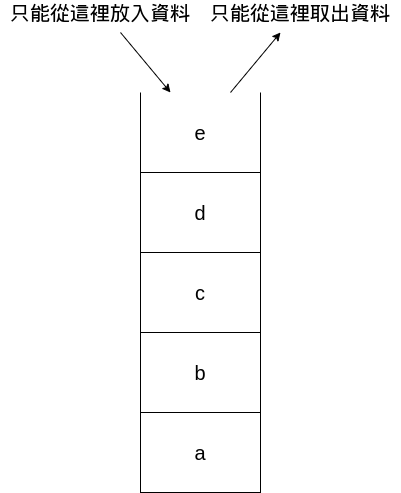### 結構

``````type stack struct {
top    *node
height int
}

type node struct {
value interface{}
below *node
}
``````

stack只需要有top的指標即可,因為不管是放入還是取出都是同一個位置

### 方法

• Push 增加一個元素
• Empty 判斷stack是否為空
• Pop 取出並移除頂端元素
• Peek 確認但不移除頂端元素

``````func (this *stack) Push(input interface{}) {
this.top = &node{input, this.top} //將原本的top指向新建立的node,此node的below為原本的top
this.height++
}

func (this *stack) Empty() bool {
return this.height == 0
}

func (this *stack) Pop() interface{} {
if this.Empty() {
return nil
}
temp := this.top //取出top
this.top = this.top.below //移動top的指標
this.height-- //修改height屬性
return temp.value
}

func (this *stack) Peek() interface{} {
if this.Empty() {
return nil
}
return this.top.value
}

func (this *stack) Prt() {
if !this.Empty() {
temp := this.top
for temp.below != nil {
fmt.Print(temp.value," ")
temp = temp.below
}
fmt.Print(temp.value," ")
}
fmt.Println()
}
``````

``````func main() {
q := stack{}
for i := 7; i < 20; i = i + 2 {
q.Push(i)
}
q.Prt()               // 19 17 15 13 11 9 7
fmt.Println(q.Peek()) // 19
fmt.Println(q.Pop())  // 19
fmt.Println(q.Pop())  // 17
fmt.Println(q.Peek()) // 15
q.Prt()               // 15 13 11 9 7
``````

## Tree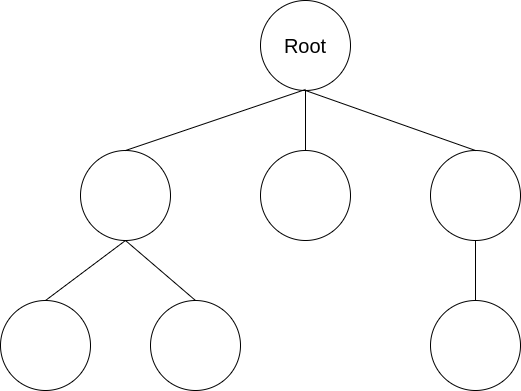Binary tree 二元樹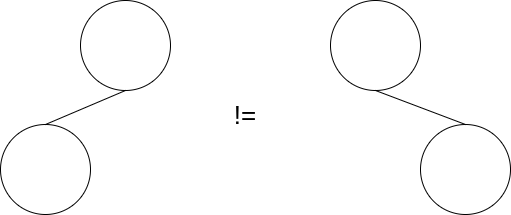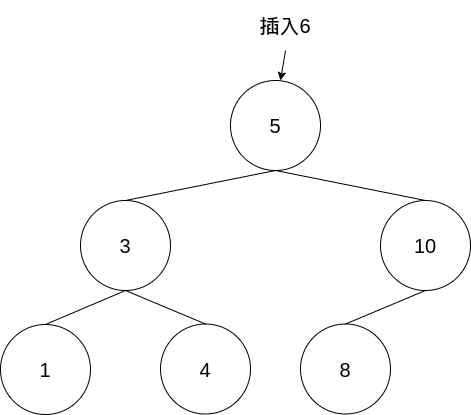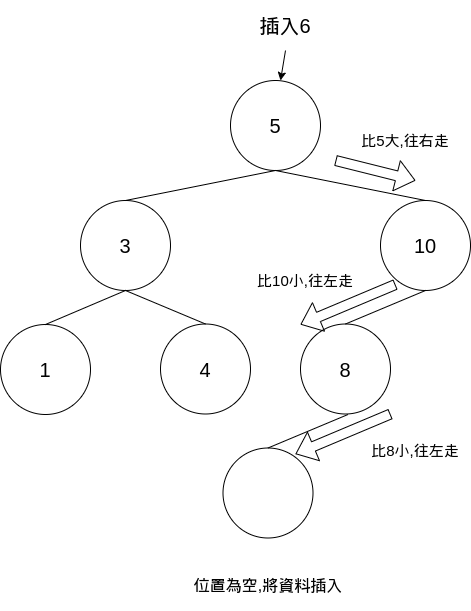### 結構

``````type TreeNode struct {
val   int
left  *TreeNode
right *TreeNode
}
``````

### 方法

• Insert 用來插入資料
• FindMax 用來查詢tree內值最大的資料(往右下角找)
• FindMin 用來查詢tree內值最小的資料(往左下角找)
• PrintInorder 用來排序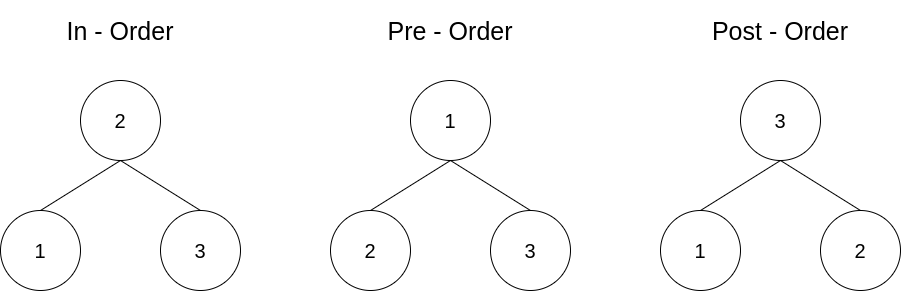``````func (t *TreeNode) Insert(value int) *TreeNode { //這裡的實作有很多種,這邊僅示範一種
if t == nil {
return &TreeNode{value, nil, nil} //若tree當前為空則建立並返回新root
}

if t.val >= value { //若輸入值小於等於root則插入在左邊
if t.left == nil {
t.left = &TreeNode{value, nil, nil}
}else{
t.left = t.left.Insert(value) //這裡要注意使用了遞迴的概念
}
return t
}

if t.val < value {
if t.right == nil {
t.right = &TreeNode{value, nil, nil}
}else{
t.right = t.right.Insert(value)
}
return t
}
return nil
}

func (t *TreeNode) FindMin() int { //這裡使用了遞迴的概念找到最左側的值
if t.left == nil {
return t.val
}
return t.left.FindMin()
}

func (t *TreeNode) FindMax() int { //這裡使用了遞迴的概念找到最右側的值
if t.right == nil {
return t.val
}
return t.right.FindMax()
}

func (t *TreeNode) PrintInorder() {
if t == nil {
return
}

t.left.PrintInorder() // 遞迴先印出左側再印出右側
fmt.Print(t.val)
t.right.PrintInorder()
}
``````

``````func (t *TreeNode) Insert(value int) error {
if t == nil {
return errors.New("Tree is nil")
}

if t.val >= value {
if t.left == nil {
t.left = &TreeNode{val: value}
return nil
}
return t.left.Insert(value)
}

if t.val < value {
if t.right == nil {
t.right = &TreeNode{val: value}
return nil
}
return t.right.Insert(value)
}
return nil
}
``````

``````type BinaryNode struct {
left  *BinaryNode
right *BinaryNode
data  int
}

type BinaryTree struct { //Tree只紀錄root的資料
root *BinaryNode
}

func (t *BinaryTree) insert(data int) *BinaryTree {
if t.root == nil { //若root為空則修改root的值
t.root = &BinaryNode{data: data, left: nil, right: nil}
} else {
t.root.insert(data) //否則則對root進行插入
}
return t
}

func (n *BinaryNode) insert(data int) {
if n == nil {
return
} else if data <= n.data {
if n.left == nil {
n.left = &BinaryNode{data: data, left: nil, right: nil}
} else {
n.left.insert(data)
}
} else {
if n.right == nil {
n.right = &BinaryNode{data: data, left: nil, right: nil}
} else {
n.right.insert(data)
}
}
}
``````

``````func main() {
deck := randCard(10)
fmt.Println(deck) //[0 6 4 7 2 9 5 8 3 1] 隨機陣列

var tree *TreeNode
for i := 0; i < len(deck); i++ {
tree = tree.Insert(deck[i])
}
fmt.Println(tree.FindMax()) // 9
fmt.Println(tree.FindMin()) // 0
tree.PrintInorder() // 0123456789
}

func randCard(num int) (pile []int) { //這個是隨機產生陣列的方法,直接照抄即可,有興趣再去研究內部的程式
rand := rand.New(rand.NewSource(time.Now().UnixNano()))
for len(pile) < num {
r := rand.Intn((num))
check := false
for i := range pile {
if pile[i] == r {
check = true
break
}
}

if !check {
pile = append(pile, r)
}
}
return
}
``````

tree還有其他種方法可以添加(比方說刪除之類的)

0